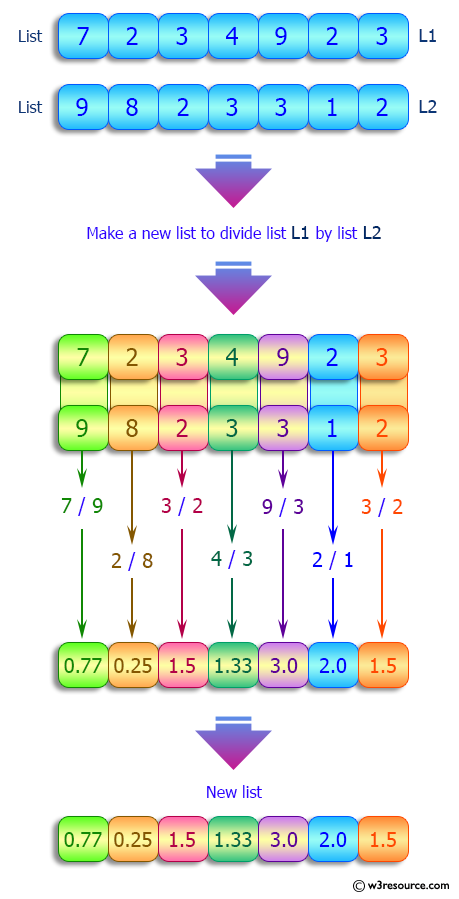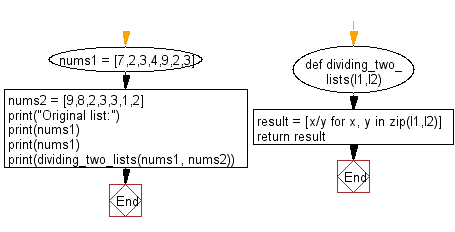﻿ Python: Create a new list dividing two given lists of numbers - w3resource# Python: Create a new list dividing two given lists of numbers

## Python List: Exercise - 176 with Solution

Write a Python program to create a new list dividing two given lists of numbers.

Sample Solution:

Python Code:

``````def dividing_two_lists(l1,l2):
result = [x/y for x, y in zip(l1,l2)]
return result
nums1 = [7,2,3,4,9,2,3]
nums2 = [9,8,2,3,3,1,2]
print("Original list:")
print(nums1)
print(nums1)
print(dividing_two_lists(nums1, nums2))
```
```

Sample Output:

```Original list:
[7, 2, 3, 4, 9, 2, 3]
[7, 2, 3, 4, 9, 2, 3]
[0.7777777777777778, 0.25, 1.5, 1.3333333333333333, 3.0, 2.0, 1.5]
```

Pictorial Presentation:Flowchart:## Visualize Python code execution:

The following tool visualize what the computer is doing step-by-step as it executes the said program:

Python Code Editor:

Have another way to solve this solution? Contribute your code (and comments) through Disqus.

What is the difficulty level of this exercise?

Test your Python skills with w3resource's quiz

﻿

## Python: Tips of the Day

Floor Division:

When we speak of division we normally mean (/) float division operator, this will give a precise result in float format with decimals.

For a rounded integer result there is (//) floor division operator in Python. Floor division will only give integer results that are round numbers.

```print(1000 // 300)
print(1000 / 300)```

Output:

```3
3.3333333333333335```